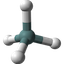What Is Molecule Structure

Q

What Is Molecule Structure?

✍: FYIcenter.com

AMolecule Structure is a graphical model of a molecule on how its atoms are bonded together, with information about atom types/sizes/locations and bound types.

Molecule Structures are 3-dimensional structures which can be visualized in different models. Two examples are list below:

Skeletal Formula - Also called line-angle formula or shorthand formula. Skeletal Formula uses line segments to represent atomic bonds, intersections/ends of line segments to represent atoms, and element symbols to identify atom types. For example, the picture below shows a Skeletal Formula of the Methane molecule: